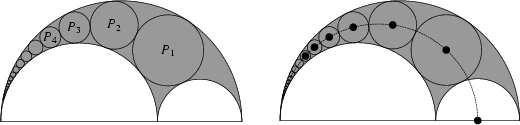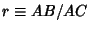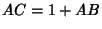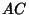## Pappus ChainIn the Arbelos, construct a chain of Tangent Circles starting with the Circle Tangent to the two small interior semicircles and the large exterior one. Then the distance from the center of the first Inscribed Circle to the bottom line is twice the Circle's Radius, from the second Circle is four times the Radius, and for theth Circle istimes the Radius. The centers of the Circles lie on an Ellipse, and the Diameter of theth Circleis ()th Perpendicular distance to the base of the Semicircle. This result was known to Pappus,who referred to it as an ancient theorem (Hood 1961, Cadwell 1966, Gardner 1979, Bankoff 1981). The simplest proof is via Inversive Geometry.

If, then the radius of theth circle in the pappus chain isThis equation can be derived by iteratively solving the Quadratic Formula generated by Descartes Circle Theorem for the radius of the Soddy Circle. This general result simplifies tofor(Gardner 1979). Further special cases whenare considered by Gaba (1940).

Ifdividesin the Golden Ratio, then the circles in the chain satisfy a number of other special properties (Bankoff 1955).

See also Arbelos, Coxeter's Loxodromic Sequence of Tangent Circles, Soddy Circles, Steiner Chain

References

Bankoff, L. The Golden Arbelos.'' Scripta Math. 21, 70-76, 1955.

Bankoff, L. Are the Twin Circles of Archimedes Really Twins?'' Math. Mag. 47, 214-218, 1974.

Bankoff, L. How Did Pappus Do It?'' In The Mathematical Gardner (Ed. D. Klarner). Boston, MA: Prindle, Weber, and Schmidt, pp. 112-118, 1981.

Gaba, M. G. On a Generalization of the Arbelos.'' Amer. Math. Monthly 47, 19-24, 1940.

Gardner, M. Mathematical Games: The Diverse Pleasures of Circles that Are Tangent to One Another.'' Sci. Amer. 240, 18-28, Jan. 1979.

Hood, R. T. A Chain of Circles.'' Math. Teacher 54, 134-137, 1961.

Johnson, R. A. Advanced Euclidean Geometry: An Elementary Treatise on the Geometry of the Triangle and the Circle. Boston, MA: Houghton Mifflin, p. 117, 1929.